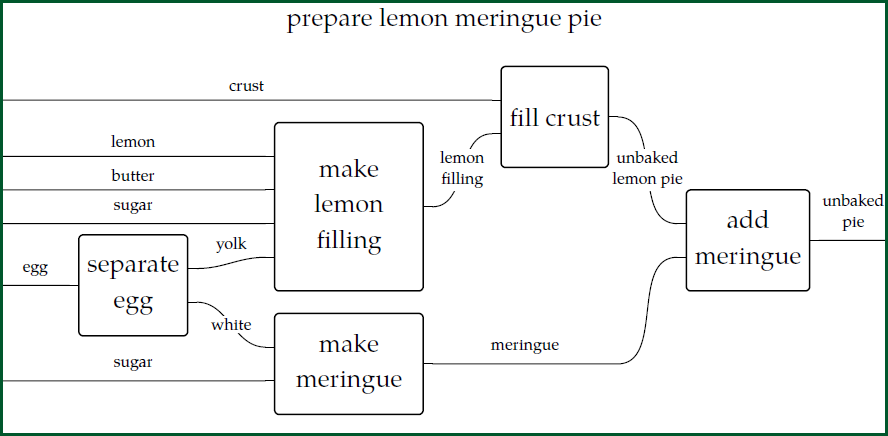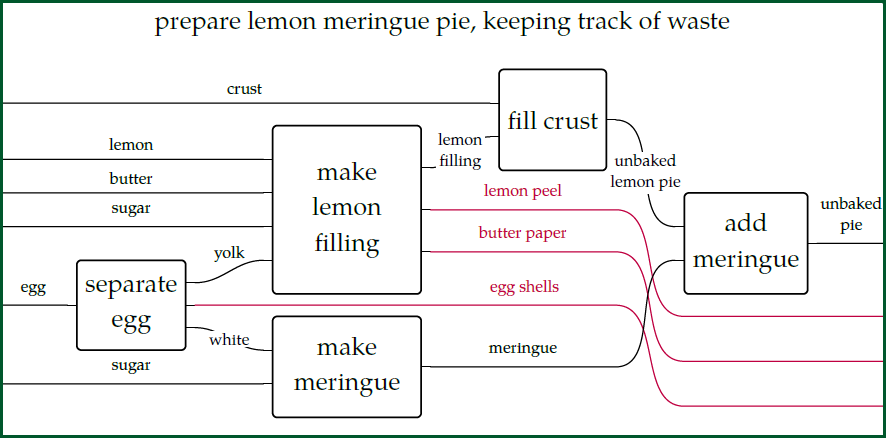We humans often ignore things we're not interested in. This is often essential. But sometimes it causes trouble. For example, global warming is caused by a civilization that has conveniently ignored a colorless, odorless gas called carbon dioxide that's a byproduct of burning carbon.

To take a humbler example, consider this wiring diagram we studied in [Lecture 25](https://forum.azimuthproject.org/discussion/2090/lecture-25-chapter-2-reaction-networks/p1):In fact this diagram ignores some of the waste products we created while making this pie. A more complete picture might look like this:Now we've added some wires in red! We're admitting that cracking eggs creates egg shells, that peeling lemons creates lemon peels, and that using sticks of butter leaves behind paper.

What's going on here, mathematically? We have _two_ stories about a process: a less complete one and a more complete one. And these are just two of many possible stories. However, all these stories are related. Category theory can help us make this more precise.

Since the above example is already rather big, let's take a simpler one. Suppose you have the ability to crack an egg into a bowl and then separate out the yolk, the white, and the egg shell. We have a set \$$S\$$ of five resources:

$S = \\{ \textrm{bowl}, \textrm{egg}, \textrm{yolk}, \textrm{white}, \textrm{egg shell} \\}$

This gives a commutative monoid consisting of combinations of these resources:

$a [\textrm{bowl}] + b [\textrm{egg}] + c[\textrm{yolk}]+ d[\textrm{white}] + e [\textrm{egg shell}]$

where \$$a,b,c,d,e \in \mathbb{N}\$$ and the square brackets are just there because they look nice. In [Lecture 25](https://forum.azimuthproject.org/discussion/2090/lecture-25-chapter-2-reaction-networks/p1) we saw that this kind of monoid is called \$$\mathbb{N}[S]\$$. But already in [Lecture 22](https://forum.azimuthproject.org/discussion/2084/lecture-22-chapter-2-symmetric-monoidal-preorders/p1) we saw how to make it into a commutative monoidal _preorder_ by choosing a reaction network and using it to define a concept of \$$\le\$$, where \$$x \le y\$$ means "we can make \$$x\$$ from \$$y\$$".

Let's choose a reaction network that describes what you can do:

$[\textrm{bowl}] + [\textrm{egg}] \to [\textrm{bowl}] + [\textrm{yolk}] + [\textrm{white}] + [\textrm{egg shell}] .$

This gives an inequality

$[\textrm{bowl}] + [\textrm{yolk}] + [\textrm{white}] + [\textrm{egg shell}] \le [\textrm{bowl}] + [\textrm{egg}]$

and we can derive a bunch more using the laws of a commutative monoidal preorder. Taking all these inequalities as true, we make \$$\mathbb{N}[S]\$$ into a commutative monoidal preorder.

But now let's ignore egg shells. And while we're at it, let's ignore the bowl! It's a "renewable resource", or "catalyst", so you might decide to take it for granted if you're in a kitchen that _has_ a bowl.

We now get a simpler set of resources:

$T = \\{ \textrm{egg}, \textrm{yolk}, \textrm{white} \\}$

We also get a simpler commutative monoid \$$\mathbb{N}[T]\$$, with elements of this form:

$b [\textrm{egg}] + c[\textrm{yolk}\ + d[\textrm{white}]$

where \$$b,c,d \in \mathbb{N}\$$. We can make this into a commutative monoidal preorder using the simpler reaction network

$[\textrm{egg}] \to [\textrm{yolk}] + [\textrm{white}] .$

which gives the inequality

$[\textrm{yolk}] + [\textrm{white}] \le [\textrm{egg}]$

and of course many others that we can derive from this using the laws of a commutative monoidal preorder.

Now for the cool part: we'll formalize the process of ignoring the waste product \$$[\textrm{egg shell}]\$$ and the renewable resource \$$[\textrm{bowl}]\$$!

I hope you can guess how it goes: I spent the last two lectures explaining [monoidal monotones](https://forum.azimuthproject.org/discussion/2095/lecture-26-chapter-2-monoidal-monotones/p1) and their [adjoints](https://forum.azimuthproject.org/discussion/2098/lecture-27-chapter-2-adjoints-of-monoidal-monotones/p1), and now we'll use those ideas.

There's function

$f : \mathbb{N}[S] \to \mathbb{N}[T]$

that forgets bowls and egg shells:

$f( a [\textrm{bowl}] + b [\textrm{egg}] + c[\textrm{yolk}] + d[\textrm{white}] + e [\textrm{egg shell}]) = b [\textrm{egg}] + c[\textrm{yolk}\ + d[\textrm{white}].$

This is the nicest possible kind of map between monoidal preorders: it's a _strict monoidal monotone!_ Remember, this means that:

1. It's monotone: if \$$x \le x'\$$ then \$$f(x) \le f(x')\$$ for all \$$x,x' \in \mathbb{N}[S]\$$.

2. It obeys \$$f(x+x') = f(x) + f(x')\$$ for all \$$x,x' \in \mathbb{N}[S]\$$.

3. It obeys \$$f(0) = 0\$$.

You can check 2 and 3 using the formula for \$$f\$$. For 1, the key thing to check is this. We know that in \$$\mathbb{N}[S]\$$ we have

$[\textrm{bowl}] + [\textrm{yolk}] + [\textrm{white}] + [\textrm{egg shell}] \le [\textrm{bowl}] + [\textrm{egg}]$

so we need to check that

$f( [\textrm{bowl}] + [\textrm{yolk}] + [\textrm{white}] + [\textrm{egg shell}]) \le f( [\textrm{bowl}] + [\textrm{egg}] )$

in \$$\mathbb{N}[T]\$$. Using the formula for \$$f\$$, this says

$[\textrm{yolk}] + [\textrm{white}] \le [\textrm{egg}] .$

But this is true: we set things up to make it true!

We actually need to check that _all_ the true inequalities in \$$\mathbb{N}[S]\$$ get sent by \$$f\$$ to true inequalities in \$$\mathbb{N}[T]\$$, but since all the inequalities in \$$\mathbb{N}[S]\$$ are derived from the one we wrote down, this is sort of obvious.

("Sort of obvious?" With a bit more category theory we could make this hand-waving argument rigorous without a boring proof by induction. But anyone who studies math or computer science needs to do a lot of boring proofs by induction, and that approach is fine too.)

Now for two very interesting puzzles. We've seen that there's a systematic way to "ignore waste products and renewable resources". But is there a systematic way to _un-ignore_ them?

That is: can we guess what a more complete description of a process is, given a less complete description? This seems like black magic. But that's what adjoints are all about: finding approximate inverses to maps that don't really have inverses! So:

**Puzzle 84.** Does the monoidal monotone \$$f : \mathbb{N}[S] \to \mathbb{N}[T] \$$ have a right adjoint? If so, what's the formula for it? Thanks to [Lecture 27](https://forum.azimuthproject.org/discussion/2098/lecture-27-chapter-2-adjoints-of-monoidal-monotones/p1) we know that this right adjoint, if it exists, is lax monoidal monotone. Is it strict monoidal monotone?

**Puzzle 85.** Does the monoidal monotone \$$f : \mathbb{N}[S] \to \mathbb{N}[T] \$$ have a left adjoint? If so, what's the formula for it? Thanks to [Lecture 27](https://forum.azimuthproject.org/discussion/2098/lecture-27-chapter-2-adjoints-of-monoidal-monotones/p1) we know that this left adjoint, if it exists, is oplax monoidal monotone. Is it strict monoidal monotone?

I'm fascinated by these puzzles, but I _haven't let myself solve them_ because I'll enjoy it more if you find the answers. Then we can philosophize about what it all means. We'll have learned something about what it means to "un-ignore" some side-effects that we'd been ignoring!

**[To read other lectures go here.](http://www.azimuthproject.org/azimuth/show/Applied+Category+Theory#Chapter_2)**